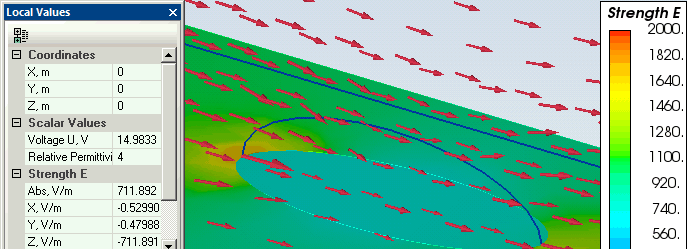QuickField

A new approach to field modelling

Main >> Applications >> Sample problems >> Dielectric ellipsoid in the uniform electric field

# Dielectric ellipsoid in a uniform electric field

QuickField simulation example

The dielectric ellipsoid is submerged into the uniform electric field. The external field vector is aligned with the ellipsoid major axis.

Problem Type
Axisymmetric problem of electrostatics.

Geometry

Given
Length along Z axis: dz = 0.05 m.
Length along X,Y axes: dx = dy = 0.02 m.
Relative permittivity of air ε0 = 1,
Relative permittivity of dielectric εr = 4,
External field strength Eext = 1 kV/m.

Find the electric field distribution inside the ellipsoid.

Solution
2D analytical solution (external field vector Eext is aligned with the ellipse Z axis):
electric field stress inside the ellipse Ez = Eext / (1+(εr - 1)·nz), where
depolarization coefficient nz = (1 - e²)/e³ * (Artanh(e) - e),
ellipse eccentricity e = sqrt(1 - dx²/dz²).

In case the external field Eext vector is aligned with one of the ellipsoid's axes, the geometry model could be constructed as 2D axisymmetric, with the model geometry presented in QuickField as an upper half of the ellipse cross-section (in general case the problem requires 3D analysis).

Results
Ellipse eccentricity e = sqrt(1 - 0.02²/0.05²) = 0.917,
depolarization coefficient nz = (1 - 0.917²)/0.917³ * (Artanh(0.917) - 0.917) = 0.135.
Uniform electric field strength inside the ellipsoid (analytical solution):
Ez = 1000 / (1 + (4 - 1)·0.135) = 711 V/m.

QuickField 2D: Ez = 712 V/m.

QuickField 3D: Ez = 711 V/m.

Electric field distribution inside and outside the dielectric ellipsoid (2D and 3D):